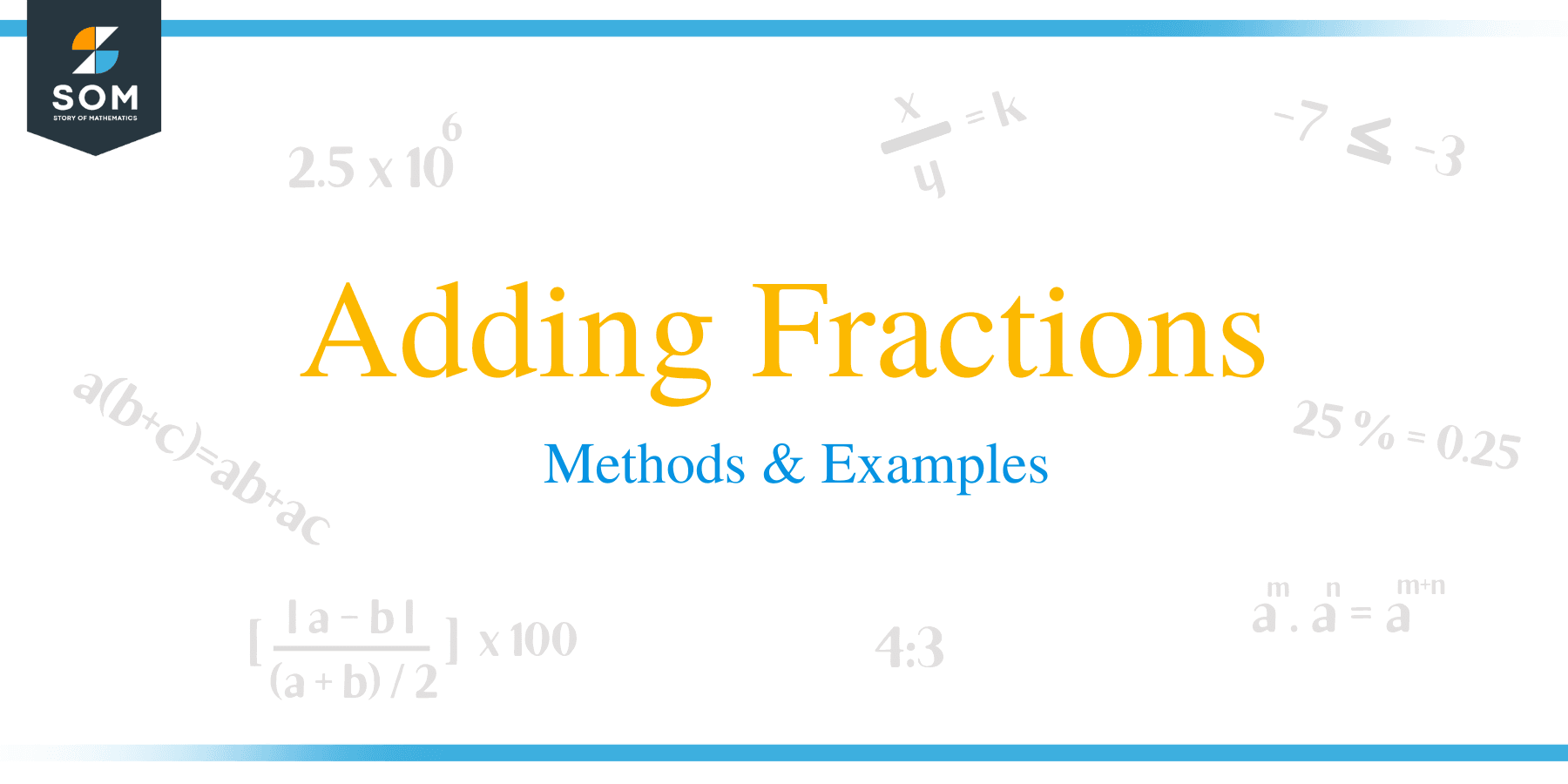# Adding Fractions – Methods & Examples

##How to Add Fractions?

To add the two fractions, the denominators of both fractions must be the same. Let’s take help of the following example to solve a simple fraction problem.

Example 1

1/2 + 1/2

We start by getting the L.C.M of the denominator which will be easy since the L.C.M of two numbers that are the same is that number.

Therefore our L.C.M. is 2

1/2+1/2 = /2

We divide the L.C.M. by the first denominator and then multiply the answer with the first numerator (This will become important when we get to addition of numbers with different denominators).

2 ÷ 2 = 1

1 × 1 = 1

We divide the L.C.M. by the second denominator and then multiply the answer with the second numerator.

2 ÷ 2 = 1

1 × 1 = 1

We then add the two results we have gotten above the L.C.M

1/2 + 1/2 = (1 + 1)/2

= 2/2

To get the answer in simplest form we will divide both the numerator and denominator by

2 to get:

1/1 = 1

Example 2

1/3+1/3

We start by getting the L.C.M of the denominator which will be easy since the L.C.M of two numbers that are the same is that number.

Therefore our L.C.M. is 3

1/3+1/3= /3

We divide the L.C.M. by the first denominator and then multiply the answer with the first numerator.

3÷3=1

1×1=1

We divide the L.C.M. by the second denominator and then multiply the answer with the second numerator.

3÷3=1

1×1=1

We then add the two results we have gotten above the L.C.M

= (1+1)/3

=2/3

### Addition of fractions having different numerators and same denominator

To understand this case, let’s see step by step solutions of the examples below.

Example 3

2/6+3/6

The L.C.M is 6 since the two denominators are the same

2/6+3/6= /6

The L.C.M which is 6 divided by the first denominator is 1, multiply 1 by the first numerator is =2

6 divided by the second denominator is 1, multiply by the second numerator is

=3

=2/6+3/6= (2+3) /6

We add the numerators above the L.C.M.

=5/6Example 4

The L.C.M is 4 since the two denominators are the same

1/4+2/4= /4

The L.C.M which is 4 divided by the first denominator which is 4 is 1, multiply 1 by the first numerator which is 1 to get =1

4 divided by the second denominator which is 4 is 1, multiply 1 by the second numerator which is 2 to get 2

We add the numerators above the L.C.M. as follows

1/4+2/4

= (1+2)/4

=3/4

### Addition of fractions having different numerators and different denominator

To understand this case, let’s see step by step solutions of the examples below.

Example 5

We find the L.C.M. of 4 and 6

 2 4 6 2 2 3 3 1 3 1 1

The L.C.M. is 2×2×3=12

=3/4+1/6= /12

Divide the L.C.M. which is 12 by the first denominator 4=3

Multiply 3 by the first numerator 3=9

Divide the L.C.M. which is 12 by the second denominator 6=2

Multiply 2 by the second numerator 1 =2

Then add the 9+2 above the L.C.M.

=3/4+1/6= (2+9) /12

=11/12

Example 6

5/7+1/3

We start by getting the L.C.M. of the two denominators 7 and 3

 3 7 3 7 7 1 1 1

The L.C.M. is 21

Divide the L.C.M. which is 21 by the first denominator which is 7 to get =3

Multiply 3 by the first numerator which is 3 to get=9

Divide the L.C.M. which is 21 by the second denominator which is 6 to get=2

Multiply 2 by the second numerator which is 1 to get =2

Then add the two results 9 and 2 above the L.C.M. to get the following

=5/7+1/3= (15+7)/21

=22/21### Practice Questions

1. What is the simplified form of $\dfrac{1}{6} + \dfrac{1}{6}$?

2. What is the simplified form of $\dfrac{1}{4} + \dfrac{1}{4}$?

3. Which of the following shows the sum of $\dfrac{2}{4}$ and $\dfrac{1}{4}$?

4. What is three-fifths added to five-sixths in simplest form?

5. Which of the following shows the simplest form when one fifth is added to three fifths?

6. If I mix $\dfrac{3}{8}$ of a liter of white paint and $\dfrac{5}{8}$ of a liter of black paint to make grey paint, how much grey paint will I make?

7. John bought $\dfrac{2}{5}$ kg of kales and $\dfrac{1}{2}$ kg of spinach. How much did the vegetables weigh together?

8. Daisy walks $\dfrac{1}{4}$ km to the market and Victor walks $\dfrac{1}{3}$ km to school. What is the total distance covered by the two pupils?